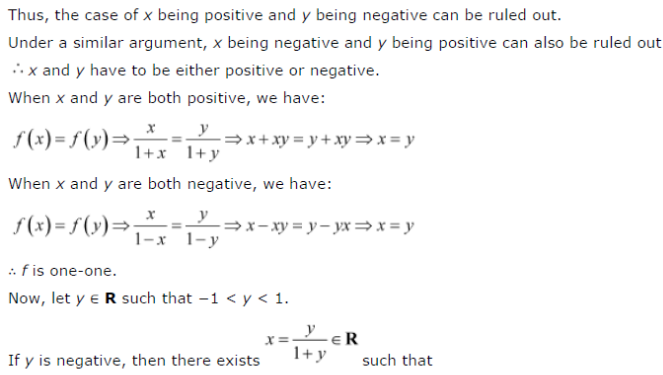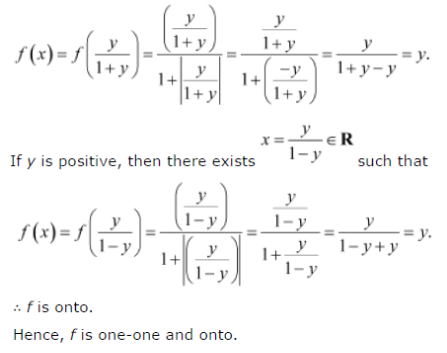# Show that the function f : R → {x ∈ R : -1 < x < 1} defined by f(x) = x/1 + |x| , x ∈ R

Show that the function f : R → {x ∈ R : -1 < x < 1} defined by f(x) = x/1 + |x| , x ∈ R, suppose f(x) = f(y), where x, y ∈ R is one-one and onto function.